# Measuring the Power of a Solar Panel

Most PV solar panels are labelled with their peak power output – this is the maximum power (measured in Watts) which that panel can generate in full sunlight. If this label is missing, or if you would like to confirm that the figures provided are accurate, you can physically measure the power of any solar panel with a quick experiment.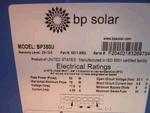### Solar Panel Power Output

The power output of a solar panel depends on the resistance of the electrical load to which it is connected. In an open circuit situation (infinite resistance) the voltage of the solar panel will be high, but no current will flow and therefore no power is generated. In a short circuit situation (zero resistance) current flows, but with zero voltage and so again no power is generated. These results all follow from Ohm’s Law which gives the relationships between voltage, current, resistance, and power.

### Measuring Solar Panel Power Output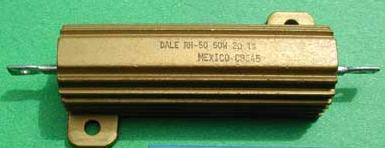A collection of power resistors of different values are required. A power resistor is a resistor which can cope with high power (and therefore heat) without being damaged. A standard 1/4 or 1/2 watt resistor will not do the job as it will overheat and be destroyed. Pictured above is a 2 Ohm resistor rated up to 50 Watts of power. (Click here for a wide range of Power Resistors.)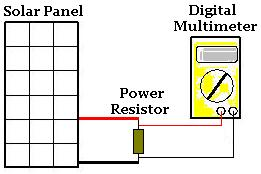Measure the voltage across the different power resistors and put the results into a table. For each measurement calculate the current (the measured voltage divided by the resistor value), and the power output (the measured voltage multiplied by the calculated current).

 Resistance (Ohms) Voltage (V) Current (A) Power (W) Open Circuit 30.9 0.00 0.00 100 28.8 0.29 8.30 50 27.0 0.54 14.58 25 22.5 0.90 20.25 3 3.1 1.03 3.20 Short Circuit 0 1.10 0.00

Above is a table of results for a typical small PV Solar Panel. Just by looking at the power column we can see that the power output is totally dependant on the value of the resistor used. The highest value of power found was with the 25 Ohm resistor at 20.25 Watts.

### Analysing the Results

If we now plot a graph of the measured voltage against the current calculated, the results are very interesting: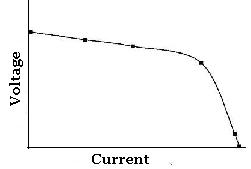The curve above is typical of all PV solar panels. The operating point for the solar panel is the mid-point of the bend in the curve. This is the point at which the solar panel generates its peak power output.

The graph below shows this operating point.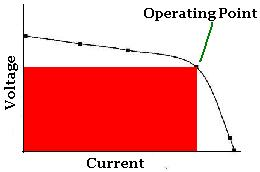Since power is given by multiplying voltage and current, the rectangle with the largest area which can be drawn under the plotted curve has its upper right hand corner at the operating point. It is impossible to fit a rectangle with a larger area under the curve.

### Calculating Peak Power Output for a Solar Panel

Multiply the values of voltage and current at the operating point to calculate the peak power for the solar panel.

### The Effect of Temperature on Peak Power Output

To find out how temperature affects the peak power output of a PV Solar Panel click here to view our article Effect of Temperature on Solar Panels.